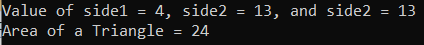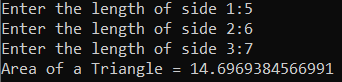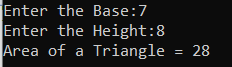# Area of Triangle in C#

## Area of Triangle Program in C# with Examples

In this article, I am going to discuss how to calculate the Area of a Triangle in C# with Examples. Please read our previous article, where we discussed the Area of Square Program in C#. Here, in this article, first, we will discuss what is triangle, then we will discuss how to calculate the area of a triangle and finally, we will see how to implement the area of triangle program in C#.

##### What is a Triangle?

It is one of the basic shapes in geometry. A Triangle is 2D closed shape, it has three sides and three angles (corners). Or, in Geometry, a triangle is a three-sided polygon that consists of three edges and three vertices. The sum of the internal angles of a triangle is equal to 180 degrees.

Semi perimeter of Triangle (S) = (side1+side2+side3)/2 or S= (a+b+c)/2

Area of a triangle (A) = √[s(s-a) (s-b) (s-c)]

Where, a, b and c are side of triangle with different length. S is the semi perimeter of triangle and A is the area of triangle

###### Example:

Input: a=5, B=6, C=7, Then s = (5 + 6 +7)/2 i.e. s = 9
Area of a triangle = √[s(s-a) (s-b) (s-c)]
=√ [9(9-5) (9-6) (9-7)]
=√ [9 × 4 × 3 × 2]
=√ [3 × 3 × 2 × 2 × 3 × 2]
=√ [32 × 22 × 3 × 2]
= 6√6 square units.

In this program, we are going to use the following Data type, and Function

1. int: Integer variables are variables that must take an integer value (0, 1, 2, …). Example: variables int n1 = 5
2. float: Floating-point numbers are used for decimal and exponential values. It is a primitive data type. Example: variables float n2 = 5.5
3. Sqrt: The Sqrt() function is used for the square root of a number. The built-in Sqrt function belongs to System.Math class. Example: squareRoot = Math.Sqrt(number);
##### Logic to Calculate the Area of Triangle in C#

To find the area of a triangle, we need to follow the below steps.

1. First, we will take variables side1, side2, side3, semiperimeter, and area.
2. Then semiperimeter will calculate by the formula of semi perimeter using the inputted value of side1, side2, and side3.
3. After calculating the semi perimeter, then we will calculate the area of the triangle by heron’s formula.
4. And the result will be store in the area variable.
##### Example: Area of Triangle Program in C#
```using System;
public class AreaOfTriangle
{
public static void Main()
{
int side1 = 4, side2 = 13, side3 = 15;
Console.WriteLine(\$"Value of side1 = {side1}, side2 = {side2}, and side2 = {side2}");
double semiperimeter = (side1 + side2 + side3) / 2;
double Area = Math.Sqrt(semiperimeter * (semiperimeter - side1) * (semiperimeter - side2) * (semiperimeter - side3));
Console.Write("Area of a Triangle = " + Area);
}
}
```
###### Output:##### Example: Area of Triangle Program in C# by taking input from the user

The following C# Program will allow the user to input the three sides of a triangle and then calculate the area and display it on the console.

```using System;
public class AreaOfTriangle
{
public static void Main()
{
Console.Write("Enter the length of side 1:");
Console.Write("Enter the length of side 2:");
Console.Write("Enter the length of side 3:");

double semiperimeter = (side1 + side2 + side3) / 2;
double Area = Math.Sqrt(semiperimeter * (semiperimeter - side1) * (semiperimeter - side2) * (semiperimeter - side3));
Console.Write("Area of a Triangle = " + Area);
}
}
```
###### Output:##### Area of Triangle using Base and Height

In some times instead of three sides of the triangle, you will get the base and height of the triangle and you will be asked to calculate the area of the triangle. The following is the formula to calculate the area of the Triangle.

Area of Triangle = (Base X Height) / 2

The following C# program allows the user to input the triangle base and height and then calculates the area and displays it in the console.

```using System;
public class AreaOfTriangle
{
public static void Main()
{
Console.Write("Enter the Base:");
Console.Write("Enter the Height:");

double Area = (Base * Height) / 2;

Console.Write("Area of a Triangle = " + Area);